SLAS429E April   2005  – June 2017

PRODUCTION DATA.

1. Features
2. Applications
3. Description
4. Revision History
5. Pin Configuration and Functions
6. Specifications
7. Detailed Description
1. 7.1 Overview
2. 7.2 Functional Block Diagram
3. 7.3 Feature Description
1. 7.3.1 DAC Section
2. 7.3.2 Power-On Reset
4. 7.4 Device Functional Modes
5. 7.5 Programming
8. Application and Implementation
1. 8.1 Application Information
2. 8.2 Typical Application
1. 8.2.1 Loop-Powered, 2-Wire, 4-mA to 20-mA Transmitter With XTR116
2. 8.2.2 Using the REF02 as a Power Supply for the DAC8551
3. 8.3 System Examples
1. 8.3.1 Microprocessor Interfacing
9. Power Supply Recommendations
10. 10Layout
11. 11Device and Documentation Support
12. 12Mechanical, Packaging, and Orderable Information

• DGK|8
• DGK|8

## 6 Specifications

### 6.1 Absolute Maximum Ratings(1)

MIN MAX UNIT
Input voltage GND –0.3 6 V
Digital input voltage GND –0.3 VDD + 0.3 V
Output voltage GND –0.3 VDD + 0.3 V
Operating temperature –40 105°C °C
Junction temperature, TJ 150°C °C
Storage temperature, Tstg –65 150 °C
Stresses beyond those listed under Absolute Maximum Ratings may cause permanent damage to the device. These are stress ratings only, which do not imply functional operation of the device at these or any other conditions beyond those indicated under Recommended Operating Conditions. Exposure to absolute-maximum-rated conditions for extended periods may affect device reliability.

### 6.2 ESD Ratings

VALUE UNIT
V(ESD) Electrostatic discharge Human-body model (HBM), per ANSI/ESDA/JEDEC JS-001(1) ±2000 V
Charged-device model (CDM), per JEDEC specification JESD22-C101(2) ±500
JEDEC document JEP155 states that 500-V HBM allows safe manufacturing with a standard ESD control process.
JEDEC document JEP157 states that 250-V CDM allows safe manufacturing with a standard ESD control process.

### 6.3 Recommended Operating Conditions

over operating free-air temperature range (unless otherwise noted)
MIN NOM MAX UNIT
Supply voltage (VDD to GND) 2.7 5.5 V
Digital input voltage (DIN, SCLK, and SYNC) 0 VDD V
VREF Reference input voltage 0 VDD V
VFB Output amplifier feedback input VOUT V
TA Operating ambient temperature –40 105 °C

### 6.4 Thermal Information

THERMAL METRIC(1) DAC8551 UNIT
DGK (VSSOP)
8 PINS
RθJA Junction-to-ambient thermal resistance 206 °C/W
RθJC(top) Junction-to-case (top) thermal resistance 44 °C/W
RθJB Junction-to-board thermal resistance 94.2 °C/W
ψJT Junction-to-top characterization parameter 10.2 °C/W
ψJB Junction-to-board characterization parameter 92.7 °C/W

### 6.5 Electrical Characteristics

VDD = 2.7 V to 5.5 V and –40°C to 105°C (unless otherwise noted)
PARAMETER TEST CONDITIONS MIN TYP MAX UNIT
STATIC PERFORMANCE(1)
Resolution 16 Bits
Relative accuracy Measured by line passing through codes 485 and 64741 at VREF = 5 V, codes 970 and 63947 at
VREF = 2.5 V
DAC8551 ±12 LSB
DAC8551A ±16 LSB
Differential nonlinearity 2.5 V ≤ VREF ≤ 5.5 V, 0°C ≤ TA ≤ 105°C ±1 LSB
4.2 V < VREF ≤ 5.5 V, -40°C ≤ TA ≤ 105°C ±1 LSB
2.5 V ≤ VREF ≤ 4.2 V, -40°C ≤ TA ≤ 0°C ±2 LSB
Zero-code error Measured by line passing through codes 485 and 64741 ±2 ±12 mV
Full-scale error ±0.05% ±0.5% FSR
Gain error Measured by line passing through codes 485 and 64741 DAC8551 ±0.02% ±0.15% FSR
DAC8551A ±0.02% ±0.2% FSR
Zero-code error drift ±5 μV/°C
Gain temperature coefficient ±1 ppm of FSR/°C
PSRR Power-supply rejection ratio RL = 2 kΩ, CL = 200 pF 0.75 mV/V
OUTPUT CHARACTERISTICS(2)
Output voltage range 0 VREF V
Output voltage settling time To ±0.003% FSR, 0200h to FD00h, RL = 2 kΩ,
0 pF < CL < 200 pF
8 10 μs
RL = 2 kΩ, CL = 50 pF 12 μs
Slew rate 1.8 V/μs
Capacitive load stability RL = ∞ 470 pF
RL = 2 kΩ 1000 pF
Code change glitch impulse 1 LSB change around major carry 0.1 nV-s
Digital feedthrough 50 kΩ series resistance on digital lines 0.1
DC output impedance At mid-code input 1 Ω
Short-circuit current VDD = 5 V 50 mA
VDD = 3 V 20
Power-up time Coming out of power-down mode, VDD = 5 V 2.5 μs
Coming out of power-down mode, VDD = 3 V 5
AC PERFORMANCE
SNR Signal-to-noise ratio BW = 20 kHz, VDD = 5 V, fOUT = 1 kHz,
1st 19 harmonics removed for SNR calculation
95 dB
THD Total harmonic distortion BW = 20 kHz, VDD = 5 V, fOUT = 1 kHz,
1st 19 harmonics removed for SNR calculation
–85 dB
SFDR Spurious-free dynamic range BW = 20 kHz, VDD = 5 V, fOUT = 1 kHz,
1st 19 harmonics removed for SNR calculation
87 dB
SINAD Signal to noise and distortion BW = 20 kHz, VDD = 5 V, fOUT = 1 kHz,
1st 19 harmonics removed for SNR calculation
84 dB
REFERENCE INPUT
Reference current VREF = VDD = 5 V 40 75 μA
VREF = VDD = 3.6 V 30 45 μA
Reference input range 0 VDD V
Reference input impedance 125
LOGIC INPUTS(2)
Input current ±1 μA
VIL Input LOW voltage 3 V ≤ VDD ≤ 5.5 V 0.3 X VDD V
2.7 V ≤ VDD < 3 V 0.1 X VDD
VIH Input HIGH voltage 3 V ≤ VDD ≤ 5.5 V 0.7 X VDD V
2.7 V ≤ VDD < 3 V 0.9 X VDD
Pin capacitance 3 pF
POWER REQUIREMENTS
VDD Supply voltage 2.7 5.5 V
IDD Supply current Normal mode, input code = 32768,
no load, does not include reference current
VDD = 3.6 V to 5.5 V,
VIH = VDD and
VIL = GND
160 250 μA
VDD = 2.7 V to 3.6 V,
VIH = VDD and
VIL = GND
140 240
All power-down modes,
VIH = VDD and VIL = GND
VDD = 3.6 V to 5.5 V 0.2 2 μA
VDD = 2.7 V to 3.6 V 0.05 2
IOUT/IDD Power efficiency ILOAD = 2 mA, VDD = 5 V 89%
Specified performance temperature –40 105 °C
Linearity calculated using a reduced codes range of 485 and 64741 at VREF = 5V, codes 970 and 63947 at VREF = 2.5V; output unloaded, 100mV headroom between reference and supply
Specified by design and characterization; not production tested.

### 6.6 Timing Characteristics

VDD = 2.7 V to 5.5 V, all specifications –40°C to 105°C (unless otherwise noted)(1)(2)
PARAMETER TEST CONDITIONS MIN TYP MAX UNIT
t1(3) SCLK cycle time VDD = 2.7 V to 3.6 V 50 ns
VDD = 3.6 V to 5.5 V 33
t2 SCLK HIGH time VDD = 2.7 V to 3.6 V 13 ns
VDD = 3.6 V to 5.5 V 13
t3 SCLK LOW time VDD = 2.7 V to 3.6 V 22.5 ns
VDD = 3.6 V to 5.5 V 13
t4 SYNC to SCLK rising edge setup time VDD = 2.7 V to 3.6 V 0 ns
VDD = 3.6 V to 5.5 V 0
t5 Data setup time VDD = 2.7 V to 3.6 V 5 ns
VDD = 3.6 V to 5.5 V 5
t6 Data hold time VDD = 2.7 V to 3.6 V 4.5 ns
VDD = 3.6 V to 5.5 V 4.5
t7 24th SCLK falling edge to SYNC rising edge VDD = 2.7 V to 3.6 V 0 ns
VDD = 3.6 V to 5.5 V 0
t8 Minimum SYNC HIGH time VDD = 2.7 V to 3.6 V 50 ns
VDD = 3.6 V to 5.5 V 33
t9 24th SCLK falling edge to SYNC falling edge VDD = 2.7 V to 5.5 V 100 ns
All input signals are specified with tR = tF = 5 ns (10% to 90% of VDD) and timed from a voltage level of (VIL + VIH) / 2.
See Figure 1.
Maximum SCLK frequency is 30 MHz at VDD = 3.6 V to 5.5 V and 20 MHz at VDD = 2.7 V to 3.6 V.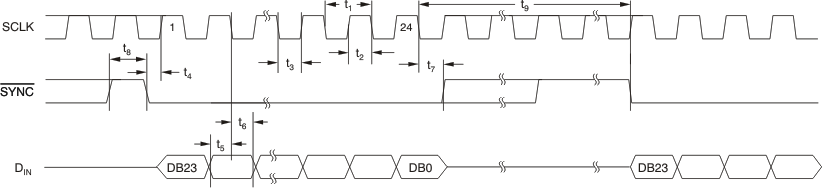Figure 1. Serial Write Operation

### 6.7 Typical Characteristics

#### 6.7.1 VDD = 5 V

At TA = 25°C (unless otherwise noted)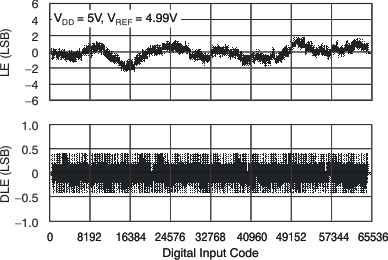Figure 2. Linearity Error and Differential Linearity Error vs Digital Input Code (–40°C)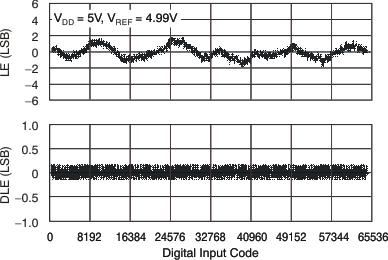Figure 4. Linearity Error and Differential Linearity Error vs Digital Input Code (105°C)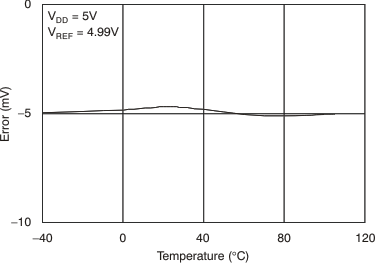Figure 6. Full-Scale Error vs Temperature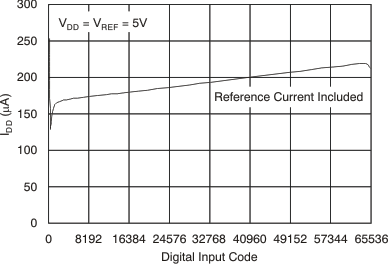Figure 8. Supply Current vs Digital Input Code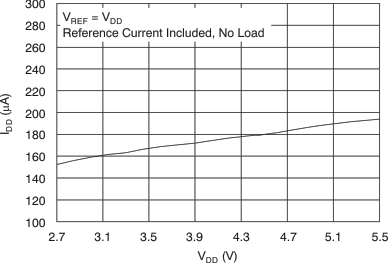Figure 10. Supply Current vs Supply Voltage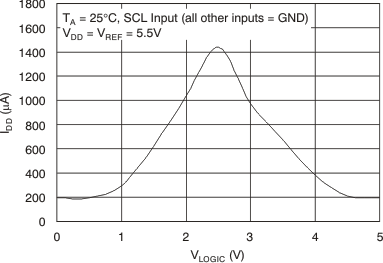Figure 12. Supply Current vs Logic Input Voltage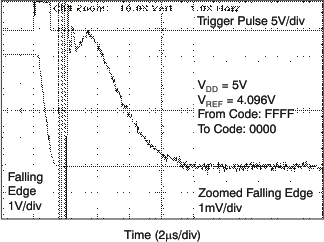Figure 14. Full-Scale Settling Time, 5-V Falling EdgeFigure 16. Half-Scale Settling Time, 5-V Falling Edge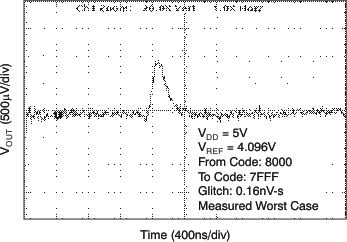Figure 18. Glitch Energy: 5-V, 1-LSB Step, Falling Edge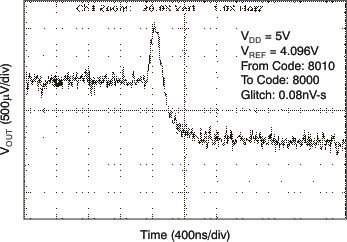Figure 20. Glitch Energy: 5-V, 16-LSB Step, Falling Edge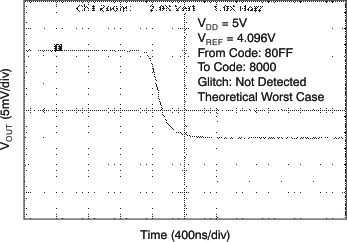Figure 22. Glitch Energy: 5-V, 256-LSB Step, Falling Edge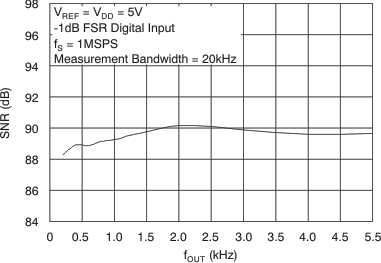Figure 24. Signal-to-Noise Ratio vs Output Frequency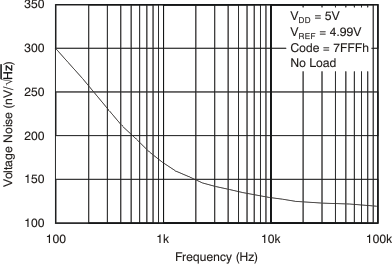Figure 26. Output Noise Density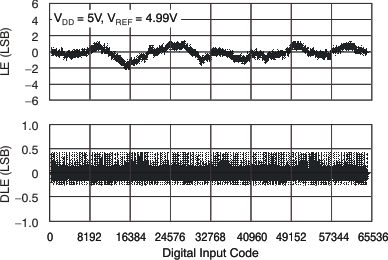Figure 3. Linearity Error and Differential Linearity Error vs Digital Input Code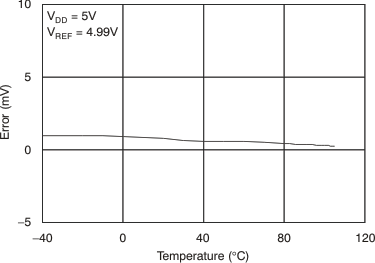Figure 5. Zero-Scale Error vs Temperature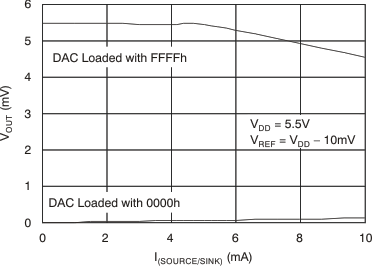Figure 7. Source and Sink Current Capability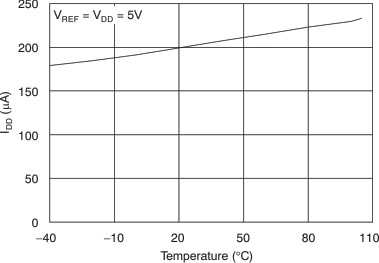Figure 9. Power-Supply Current vs Temperature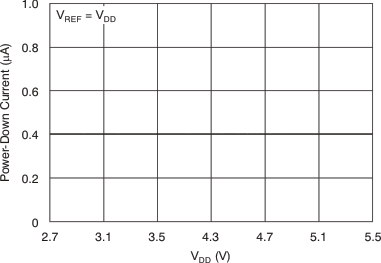Figure 11. Power-Down Current vs Supply Voltage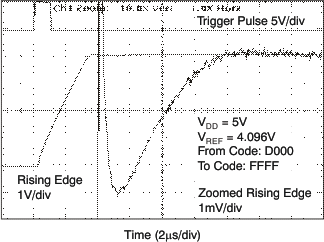Figure 13. Full-Scale Settling Time, 5-V Rising Edge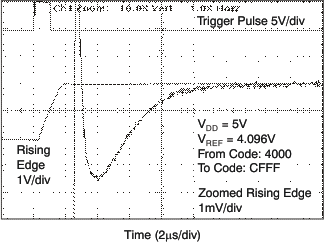Figure 15. Half-Scale Settling Time, 5-V Rising Edge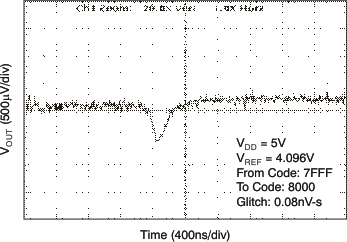Figure 17. Glitch Energy: 5-V, 1-LSB Step, Rising Edge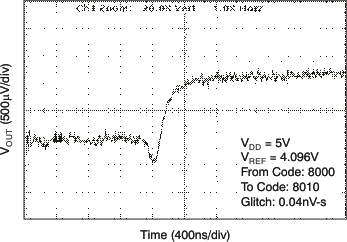Figure 19. Glitch Energy: 5-V, 16-LSB Step, Rising Edge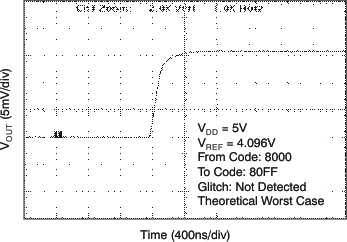Figure 21. Glitch Energy: 5-V, 256-LSB Step, Rising EdgeFigure 23. Total Harmonic Distortion vs Output Frequency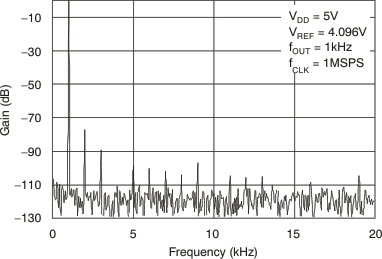Figure 25. Power Spectral Density

#### 6.7.2 VDD = 2.7 V

At TA = 25°C (unless otherwise noted)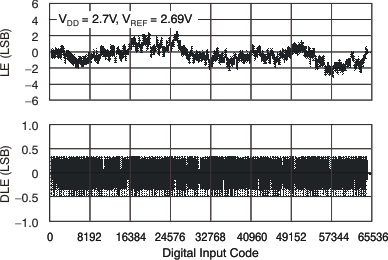Figure 27. Linearity Error and Differential Linearity Error vs Digital Input Code (–40°C)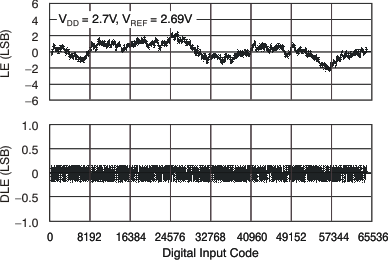Figure 29. Linearity Error and Differential Linearity Error vs Digital Input Code (105°C)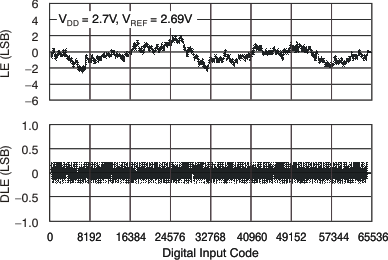Figure 28. Linearity Error and differential Linearity Error vs Digital Input CodeFigure 30. Zero-Scale Error vs Temperature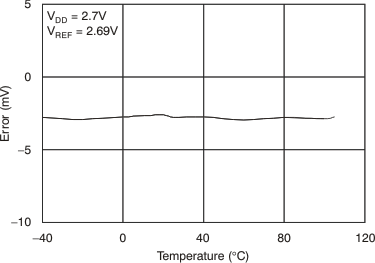Figure 31. Full-Scale Error vs Temperature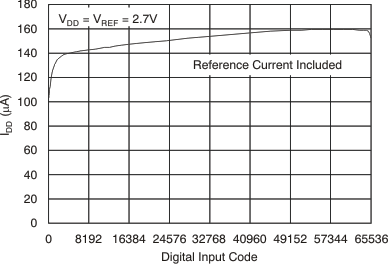Figure 33. Supply Current vs Digital Input Code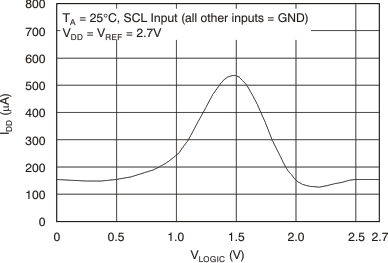Figure 35. Supply Current vs Logic Input Voltage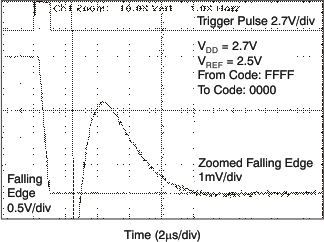Figure 37. Full-Scale Settling Time: 2.7-V Falling Edge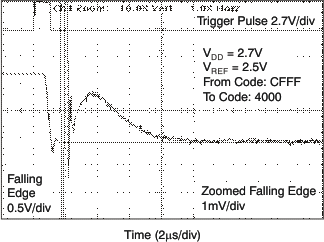Figure 39. Half-Scale Settling Time: 2.7-V Falling Edge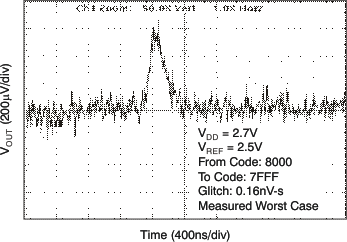Figure 41. Glitch Energy: 2.7-V, 1-LSB Step, Falling EdgeFigure 43. Glitch Energy: 2.7-V, 16-LSB Step, Falling Edge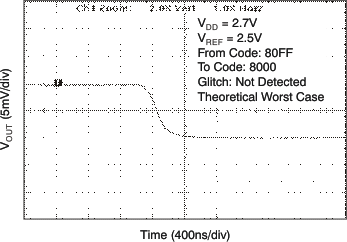Figure 45. Glitch Energy: 2.7-V, 256-LSB Step, Falling Edge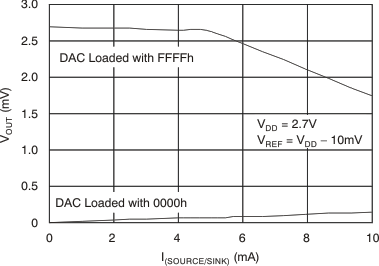Figure 32. Source and Sink Current Capability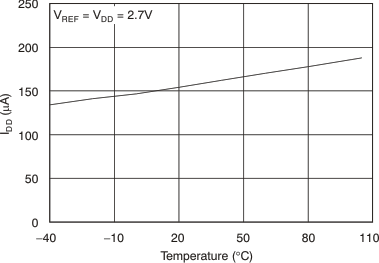Figure 34. Power-Supply Current vs Temperature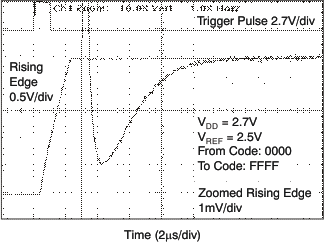Figure 36. Full-Scale Settling Time: 2.7-V Rising EdgeFigure 38. Half-Scale Settling Time: 2.7-V Rising Edge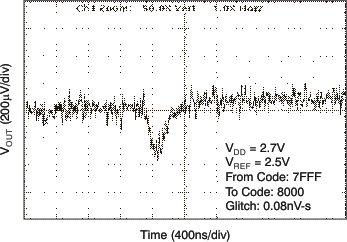Figure 40. Glitch Energy: 2.7-V, 1-LSB Step, Rising Edge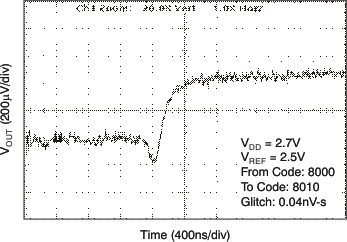Figure 42. Glitch Energy: 2.7-V, 16-LSB Step, Rising EdgeFigure 44. Glitch Energy: 2.7-V, 256-LSB Step, Rising Edge